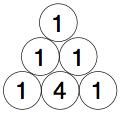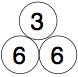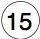#### You may also like### Prompt Cards

These two group activities use mathematical reasoning - one is numerical, one geometric.### Consecutive Numbers

An investigation involving adding and subtracting sets of consecutive numbers. Lots to find out, lots to explore.### Exploring Wild & Wonderful Number Patterns

EWWNP means Exploring Wild and Wonderful Number Patterns Created by Yourself! Investigate what happens if we create number patterns using some simple rules.

# Build it up More

##### Age 7 to 11 Challenge Level:
This is written as a follow-on from Build it Up.

You could extend what you did in Build it Up by thinking of it in 3D!

The circles would then be spheres.
Each numbered sphere would then sit on three underneath and the whole thing would end up being a triangular based pyramid.

So there would be six numbers (in an equilateral triangle) at the bottom, like this as an example:Then the next layer up would be:So the top layer would be:You can explore finding a triangle of six numbers (we don't use zero!), add them in threes, to give a triangle of three numbers and those three numbers adding to 15.